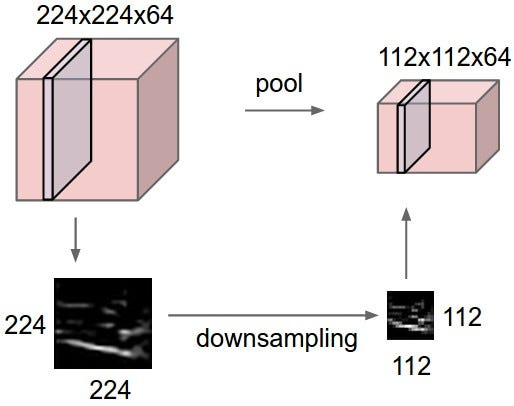# WHY POOLING IS NOT THE ANSWER TO EVERY PROBLEM

Source: Deep Learning on Medium

Pooling Function is very popular in Convolutional Neural Network. By using these functions, in many cases, the performance of a Machine Learning model can be increased dramatically. However sometimes these functions, instead of increasing the performance, decreases the performance of the Machine Learning model. In this post, I’ll discuss in detail about Why Pooling Function doesn’t work always?

In a typical Convolution Neural Network, pooling is a very important step. In CNN, the first layer performs a convolutional operation, the second layer is a ReLu layer, which introduces non-linearity. And the final layer is the pooling layer.

There are different types of polling. Max Pooling is the most popular type of pooling. Average Pooling and L2 norm pooling are some other widely used pooling functions.

“The pooling operation used in convolutional neural networks is a big mistake and the fact that it works so well is a disaster.”
—
Geoffrey Hinton

### TO UNDERSTAND WHY POOLING FUNCTION DOESN’T WORK ALWAYS, FIRST WE NEED TO UNDERSTAND WHAT POOLING FUNCTION DO!!!

Pooling Function in a CNN performs several actions. Pooling Function reduces the size of the output of the Convolution operation. By reducing the size of the output, it makes learning simple and computationally inexpensive.

Another advantage of Pooling Function is that it reduces overfitting in the network.Pooling Function reducing the size of the output of the convolution layer

Pooling Function also helps in extracting the dominant features in which are rotational and positional invariant and thus makes the process of learning simpler.

### NOW LET’S TALK ABOUT THE PROBLEMS WITH POOLING FUNCTION

The problem with pooling function is that it makes the representation approximately invariant to small changes. In other words, most of the output of the Pooling Function remains the same if we change the input of the pooling function slightly.

Thus in many cases, where we want the network to react to small changes, pooling decreases the performance of the network. In these cases, we should avoid using Pooling Function.

At the same time, in many cases, where we want the network to be invariant to small changes, Pooling Function increases the performance drastically.

For example, in images classification, Pooling Function brilliantly works and increases the accuracy of the model.

### Conclusion

In most of the cases, especially in Computer Vision, Pooling Function increases the accuracy of the model. But in some cases, where we want our model to be super sensitive towards small changes, we should avoid Pooling Function.

Thank you for reading this post. If you want to contact me for any reason, please send a message to me at twitter. I’m available @imdeepmind.

Images used in this post are from pixabay.com, Stanford University, and the deep learning book